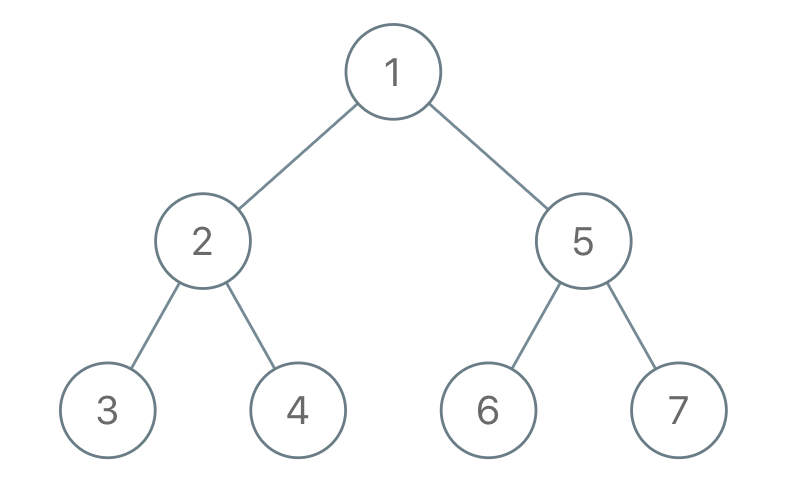## 1028. Recover a Tree From Preorder Traversal

We run a preorder depth-first search (DFS) on the `root` of a binary tree.

At each node in this traversal, we output `D` dashes (where `D` is the depth of this node), then we output the value of this node.  If the depth of a node is `D`, the depth of its immediate child is `D + 1`.  The depth of the `root` node is `0`.

If a node has only one child, that child is guaranteed to be the left child.

Given the output `S` of this traversal, recover the tree and return its `root`.

Example 1:```Input: S = "1-2--3--4-5--6--7"
Output: [1,2,5,3,4,6,7]
```

Example 2:```Input: S = "1-2--3---4-5--6---7"
Output: [1,2,5,3,null,6,null,4,null,7]
```

Example 3:```Input: S = "1-401--349---90--88"
Output: [1,401,null,349,88,90]
```

Constraints:

• The number of nodes in the original tree is in the range `[1, 1000]`.
• `1 <= Node.val <= 109`

## Rust Solution

``````struct Solution;
use rustgym_util::*;
use std::iter::Peekable;
use std::str::Chars;
use std::vec::IntoIter;

enum Tok {
N(i32),
D(usize),
}

trait Preorder {
fn parse(it: &mut Peekable<IntoIter<Tok>>, depth: usize) -> TreeLink;
fn parse_root(it: &mut Peekable<IntoIter<Tok>>) -> TreeLink;
}

fn parse(it: &mut Peekable<IntoIter<Tok>>, depth: usize) -> TreeLink {
if let Some(&Tok::D(d)) = it.peek() {
if d == depth {
it.next();
if let Some(Tok::N(n)) = it.next() {
} else {
None
}
} else {
None
}
} else {
None
}
}

fn parse_root(it: &mut Peekable<IntoIter<Tok>>) -> TreeLink {
if let Some(Tok::N(n)) = it.next() {
} else {
None
}
}
}

impl Solution {
fn recover_from_preorder(s: String) -> TreeLink {
let toks: Vec<Tok> = Self::parse_tokens(&mut s.chars().peekable());
}

fn parse_tokens(it: &mut Peekable<Chars>) -> Vec<Tok> {
let mut toks: Vec<Tok> = vec![];
while let Some(c) = it.next() {
match c {
'-' => {
let mut d = 1;
while let Some('-') = it.peek() {
it.next();
d += 1;
}
toks.push(Tok::D(d));
}
'0'..='9' => {
let mut n = (c as u8 - b'0') as i32;
while let Some('0'..='9') = it.peek() {
n *= 10;
n += (it.next().unwrap() as u8 - b'0') as i32;
}
toks.push(Tok::N(n));
}
_ => {}
}
}
toks
}
}

#[test]
fn test() {
let s = "1-2--3--4-5--6--7".to_string();
let res = tree!(
1,
tree!(2, tree!(3), tree!(4)),
tree!(5, tree!(6), tree!(7))
);
assert_eq!(Solution::recover_from_preorder(s), res);
let s = "1-2--3---4-5--6---7".to_string();
let res = tree!(
1,
tree!(2, tree!(3, tree!(4), None), None),
tree!(5, tree!(6, tree!(7), None), None)
);
assert_eq!(Solution::recover_from_preorder(s), res);
let s = "1-401--349---90--88".to_string();
let res = tree!(1, tree!(401, tree!(349, tree!(90), None), tree!(88)), None);
assert_eq!(Solution::recover_from_preorder(s), res);
}
``````

Having problems with this solution? Click here to submit an issue on github.